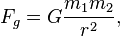# Newton's law of universal gravitation facts for kids

Kids Encyclopedia Facts
(Redirected from Law of gravitation)

Newton's universal law of gravitation is a physical law that describes the attraction between two objects with mass. It is talked about in Isaac Newton's Philosophiae Naturalis Principia Mathematica. The law is part of classical mechanics.

The formula is$F_{g} = G \frac{m_1 m_2}{r^2},$

In this equation:

• Fg is the total gravitational force between the two objects.
• G is the gravitational constant.
• m1 is the mass of the first object.
• m2 is the mass of the second object.
• r is the distance between the centres of the objects.

In SI units, Fg is measured in newtons (N), m1 and m2 in kilograms (kg), r in metres (m), and the constant G is approximately equal to 6.674×10−11 N m2 kg−2.

## Images for kidsNewton's law of universal gravitation Facts for Kids. Kiddle Encyclopedia.